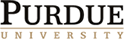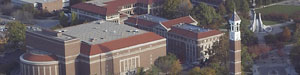Fall 2008
Time and place: Thursdays 3:30-4:30 in REC 117

Organizer: Ralph Kaufmann. Contact organizer.

Abstracts

09/04: James McClure (Purdue): Cup product in intersection cohomology .
Abstract: Joint with Greg Friedman. Very little work has been done on intersection cohomology (as opposed to intersection homology). It's natural to ask whether there's a cup product in intersection cohomology. This isn't obvious, because there is no Alexander-Whitney map. But Greg and I have shown that one way of defining the cup product in ordinary cohomology does generalize to intersection cohomology.

09/11 and 09/18: Ben Walter (Purdue): Cooperads and Coalgebras, or What is a Cofree Coalgebra? (Part 1 and 2)
More details on the constructions will be given in the second part of the talk.

09/25: Ralph Kaufmann (Purdue): From Graphs to Actions
Abstract: We explain how graphs on surfaces yield multilinear maps or better correlators for algebras with a cyclic trace via a universal formula. In particular applying this formula in the case of a Frobenius algebra and restricting the graphs, we obtain string topology type operations or operations of a graph complex computing the cohomology moduli spaces. If time permits, we discuss quantum deformations of this setup.

10/16: Ben Walter (Purdue): A new model for Harrison homology
Abstract:
Classically the Harrison complex is given by quotienting the Hochschild complex of a differential graded commutative algebra by the subcomplex of shuffles. The first Eulerian idempotent gives a homotopy splitting of the quotient map from the Hochschild complex to the Harrison complex, so Harrison homology can be used to recover part of Hochschild homology. Also classically, Harrison homology is the Andre-Quillen homology theory for commutative rings. Koszul duality theory for operads then implies that Harrison homology should have the structure of a Lie coalgebra. I will give a new model for Harrison homology using coalgebras of graphs in which the Lie coalgebra structure is clear. The model will also come with an easy way to distinguish when two elements in the Harrison complex are different which I will use to give extremely simple proofs of standard Harrison theory propositions. The model I propose is analogous to expressing a Lie algebra as a quotient of a non-associative algebra -- the classical construction is analogous to expressing a Lie algebra as a subalgebra of a non-commutative algebra with the commutator bracket.

11/06: Vigleik Angeltveit (U of Chicago): Uniqueness of Morava K-theory.
Classical obstruction theory seemingly produces uncountably many
A-infinity structures on the Morava K-theory spectrum K(n). We show
that these A-infinity structures are all equivalent, using a
Bousfield-Kan spectral sequence converging to the homotopy groups of
the moduli space of A-infinity ring spectra equivalent to K(n). This
spectral sequence has infinitely many differentials, and to show that
all the relevant classes die we study the connective Morava K-theory
spectrum k(n) and use the theory of Postnikov towers and S-algebra
k-invariants developed by Dugger and Shipley.

11/13: Stephan Stolz (Notre Dame): Supersymmetric Euclidean field theories and generalized cohomology
This is a report of ongoing joint work with Peter Teichner (Berkeley). Elaborating Segal's axiomatic approach to conformal field theories, we define supersymmetric Euclidean field theories over a manifold X. It turns out that the set of concordance classes of such field theories over X of dimension d is in bijective correspondence to the cohomology of X (with complex coefficients) for d=0 and to the K-theory  of X for d=1. We speculate that for d=2 we obtain the "Topological Modular Form theory" of Hopkins-Miller. Evidence is provided by our result that the partition function of a supersymmetric Euclidean field theory of dimension 2 is a weakly  holomorphic integral modular form.

11/20: Tom Fiore: A Thomason Model Structure on nFoldCat
Abstract: When are two categories the same? One possible notion of weak equivalence is an equivalence of categories, another is a functor whose nerve is a weak homotopy equivalence of simplicial sets. As is well known, these distinct notions of weak equivalence between categories have been encoded in model structures by Joyal-Tierney and Thomason. One can ask the same question for Ehresmann's internal categories in Cat: when are two double categories the same? Or, more generally, when are two n-fold categories the same? There are several reasonable notions of weak equivalence. One uses the nerve: an n-fold functor is a weak equivalence if and only if the diagonal of its nerve is a weak equivalence of simplicial sets. Our Theorem states that such n-fold functors form the weak equivalences of a model structure on nFoldCat, and that this model structure is Quillen equivalent to the standard structure on simplicial sets.
There are several reasons for interest in this topic. A double category is good context in which to embed two types of morphisms: ring homomorphisms and bimodules form the morphisms of a double category, as do cobordisms and diffeomorphisms. A double category is also a good place to treat base change. The Thomason model structure is of interest because of Quillen's Theorems A and B, as well as Thomason's result on certain nerves of categories as models for certain homotopy colimits.
This is joint work with Simona Paoli.

12/04: Tony Elmendorf: Permutative categories, multicategories, and algebraic K-theory
Abstract: One of the standard methods for producing algebraic K-theory spectra involves a construction that associates a spectrum to any permutative category. In one of his last papers, Robert Thomason showed that this construction produces all connective spectra, as well as all homotopy classes of maps between them, and claimed that he could produce a symmetric monoidal multiplicative structure on permutative categories that models the multiplicative structure of connective stable homotopy. In this talk I will describe joint work with Mike Mandell in which we give a symmetric monoidal category that is also bicomplete, which maps multiplicatively to connective spectra and in which permutative categories embed. The trick is to look at more general structures than permutative categories, called multicategories (also known as colored operads) and show that the construction of the associated spectrum extends, and actually has nicer properties than the construction used by Thomason and others